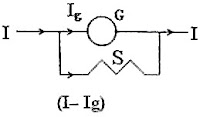## Thursday, June 21, 2007

### Two KEAM (Engineering) 2007 Questions from Electrodynamics

The following multiple choice questions appeared in Kerala Engineering Entrance 2007 question paper:

(1) A conducting rod of 1 m length and 1 kg mass is suspended by two vertical wires through its ends. An external magnetic field of 2 T is applied normal to the rod. Now the current to be passed through the rod so as to make the tension in the wires zero is [Take g = 10 m s–2]

(a) 0.5 A (b) 15 A (c) 5 A (d) 1.5 A (e) 2.5 A

The magnetic force (F) on the rod is given by

F = ILB sinθ where ‘I’ is the current, ‘L’ is the length of the rod, ‘B’ is the magnetic field andθ’ is the angle between the magnetic field and the rod. Since θ = 90º,

F = ILB = I×1×2 = 2I.

The tension in the wires will be zero when the magnetic force balances the weight of the rod.

Therefore, 2I = mg = 1×10 from which I = 5 A.

[It would have been better to mention the magnetic field to be horizontal, in addition to being normal to the rod]

(2) A galvanometer of resistance 20 Ω shows a deflection of 10 divisions when a current of 1 mA is passed through it. If a shunt of 4 Ω is connected and there are 50 divisions on the scale, the range of the galvanometer is

(a) 1 A (b) 3 A (c) 10 mA (d) 30 A (e) 30 mA

This question basically pertains to the conversion of a galvanometer in to an ammeter. Since there are 50 divisions on the scale, the current (Ig) required for full scale deflection is 5 mA.When the shunt ‘S’ is connected, the maximum current (‘range of galvanometer’, as mentioned in the question) that can be passed into the system (I) is given by

IgG = (I–Ig)S where ‘G’ is the resistance of the galvanometer.

Therefore, I = Ig(G+S)/S = 5×10–3(20+4)/4 = 30×10–3 A = 30 mA.

[You can solve this problem in no time like this: Since the shunt is 1/5th of the resistance of the galvanometer, it will pass five times the current through the galvanometer. So, the total (maximum) current that can be passed in to the system is six times Ig, which is 6×5 mA = 30 mA]